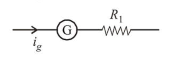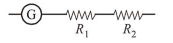# A galvanometer of resistance G is converted into

Question:

A galvanometer of resistance $G$ is converted into a voltmeter of ragne $0-1 \mathrm{~V}$ by connecting a resistance $\mathrm{R}_{1}$ in series with it. The additional resistance $R_{1}$ in series with it. The additional resistance that should be connected in series with $R_{1}$ to increase the range of the voltmeter to $0-2 \mathrm{~V}$ will be:

1. (1) $\mathrm{G}$

2. (2) $\mathrm{R}_{1}$

3. (3) $\mathrm{R}_{1}-\mathrm{G}$

4. (4) $\mathrm{R}_{1}+\mathrm{G}$

Correct Option: 4

Solution:

(4) Galvanometer of resistance (G) converted into a voltmeter of range $0-1 \mathrm{~V}$.$V=1=i_{g}\left(G+R_{1}\right)$                         ...(1)

To increase the range of voltmeter $0-2 \mathrm{~V}$$2=i_{g}\left(R_{1}+R_{2}+G\right)$                             ...(2)

Dividing eq. (i) by (ii),

$\Rightarrow \frac{1}{2}=\frac{G+R_{1}}{G+R_{1}+R_{2}}$

$\Rightarrow G+R_{1}+R_{2}=2 G+2 R_{1}$

$\therefore R_{2}=G+R_{1}$